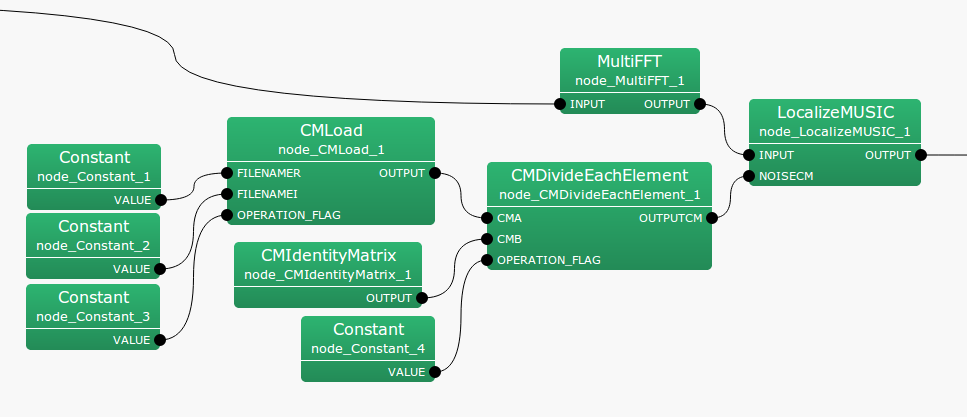## 6.2.6 CMDivideEachElement

### 6.2.6.1 Module Overview

Performs component-wise division of two sound source correlation matrices.

None.

### 6.2.6.3 Usage

In what case is the node used?

The calculation node of the correlation matrix is the one created for the sound source from CMMakerFromFFT , CMMakerFromFFTwithFlag , and has the function of dividing each component.

Typical Examples

Figure. 6.22 shows the usage example of CMDivideEachElement  node. The CMA input terminal is connected to a correlation matrix calculated from CMMakerFromFFT  or CMMakerFromFFTwithFlag , etc. (type is Matrix<complex<float> >  type, but to handle a correlation matrix, convert the three dimensional complex array to a two dimensional complex array and then output). Like CMA, the CMB input terminal also connects to the correlation matrix. At the time of division, CMA ./ CMB is calculated, where ./ shows component-wise division. OPERATION_FLAG is int  type, or bool  type input, and controls when the correlation matrix division is calculated.Figure 6.22: Network Example using CMDivideEachElement

### 6.2.6.4 I/O and property setting of the node

Table 6.22: Parameter list of CMDivideEachElement
 Parameter Type Default Unit Description FIRST_FRAME_EXECUTION false Selection of first frame execution only ENABLE_DEBUG false ON/OFF of debugging information output

Input

CMA

: Matrix<complex<float> >  type. A correlation matrix for each frequency bin. The $M$-th order complex square array correlation matrix inputs $NFFT/2 + 1$ items. Matrix<complex<float> >  contains rows corresponding to frequency ($NFFT/2 + 1$ rows), and columns containing the complex correlation matrix ($M * M$ columns across).

CMB

: Matrix<complex<float> >  type. Same as CMA.

OPERATION_FLAG

: int  type or bool  type. Only when this input terminal is 1 or when true, calculation of the correlation matrix is performed.

Output

OUTPUTCM

: Matrix<complex<float> >  type. The correlation matrix equivalent to CMA ./ CMB after division is output.

Parameter

FIRST_FRAME_EXECUTION

: bool  type. Default value is false. When true, OPERATION_FLAG is always 0. Even when it is false, the operation is performed only on the first frame.

ENABLE_DEBUG

: bool  type. Default value is false. When true, output the frame number calculated to the standard output, during dividing the correlation matrix division.

### 6.2.6.5 Module Description

Performs component-wise division of the two correlation matrices. Note that it is not a matrix division of the correlation matrix. The correlation matrix is a complex three-dimensional array of size $k \times M \times M$ and the division $k \times M \times M$ times is performed as follows. Here, $k$ is the number of frequency bins ($k = NFFT/2 + 1$), and $M$ is the number of channels in the input signal.


OUTPUTCM = zero_matrix(k,M,M)

calculate{

IF OPERATION_FLAG
FOR i = 1 to k
FOR i = 1 to M
FOR i = 1 to M

OUTPUTCM[i][j][k] = CMA[i][j][k] / CMB[i][j][k]

ENDFOR
ENDFOR
ENDFOR
ENDIF

}


The matrix that is output from the OUTPUTCM terminal is initialized as a zero matrix, and maintains the final operational result from this point onward.